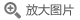## 二阶抛物微分方程(修订版)(英文版)

• 定价： ￥99
• ISBN：9787519264215
• 开 本：16开 平装
• 作者：(美)G.M.利伯曼
• 立即节省：
• 2019-09-01 第1版
• 2019-09-01 第1次印刷### 导语### 内容提要1977年，德国Springer出版了《二阶椭圆偏微分方程》（Elliptic Partial Differential Equations of Second Order, D. Gilbarg, S. Trudinger）。20年之后的1996年，G. M. Lieberman撰写了《二阶抛物微分方程》，成为《二阶椭圆偏微分方程》的姊妹篇。几十年来，这两部书的均成为受读者欢迎的经典教科书。

### 目录

PREFACE
PREFACE TO REVISED EDITION
Chapter Ⅰ  INTRODUCTION
1.Outline of this book
2.Further remarks
3.Notation
Chapter Ⅱ  MAXIMUM PRINCIPLES
Introduction
I.The weak maximum principle
2.The strong maximum principle
3.A priori estimates
Notes
Exercises
Chapter Ⅲ  INTRODUCTION TO THE THEORY OF WEAK SOLUTIONS
Introduction
1.The theory of weak derivatives
2.The method of continuity
3.Problems in small balls
4.Global existence and the Perron process
Notes
Exercises
Chapter Ⅳ  HOLDER ESTIMATES
Introduction
1.Ho1der continuity
2.Campanato spaces
3.Interior estimates
4.Estimates near a flat boundary
5.Regularized distance
6.Intermediate Schauder estimates
7.Curved boundaries and nonzero boundary data
8.Two special mixed problems
Notes
Exercises
Chapter Ⅴ  EXISTENCE, UNIQUENESS AND REGULARITY OF SOLUTIONS
Introduction
1.Uniqueness of solutions
2.The Cauchy-Dirichlet problem with bounded coefficients
3.The Cauchy-Dirichlet problem with unbounded coefficients
4.The oblique derivative problem
Notes
Exercises
Chapter Ⅵ  FURTHER THEORY OF WEAK SOLUTIONS
Introduction
1.Notation and basic results
2.Differentiability of weak solutions
3.Sobolev inequalities
4.Poincarf's inequality
5.Global boundedness
6.Local estimates
7.Consequences of the local estimates
8.Boundary estimates
9.More Sobolev-type inequalities
10.Conormal problems
11.A special mixed problem
12.Solvability in H61der spaces
13.The parabolic DeGiorgi classes
Notes
Exercises
Chapter Ⅶ  STRONG SOLUTIONS
Introduction
1.Maximum principles
2.Basic results from harmonic analysis
3.Lp estimates for constant coefficient divergence structure equations
4.Interior Lp estimates for solutions of nondivergence form constant coefficient equations
5.An interpolation inequality
6.Interior Lp estimates
7.Boundary and global estimates
8.Wp2,1 estimates for the oblique derivative problem
9.The local maximum principle
10.The weak Harnack inequality
11.Boundary estimates
Notes
Exercises
Chapter Ⅷ  FIXED POINT THEOREMS AND THEIR APPLICATIONS
Introduction
1.The Schauder fixed point theorem
2.Applications of the Schauder theorem
3.A theorem of Caristi and its applications
Notes
Exercises
Chapter Ⅸ  COMPARISON AND MAXIMUM PRINCIPLES
Introduction
I.Comparison principles
2.Maximum estimates
3.Comparison principles for divergence form operators
4.The maximum principle for divergence form operators
Notes
Exercises
Introduction
1.The boundary gradient estimate in general domains
2.Convex-increasing domains
3.The spatial distance function
4.Curvature conditions
5.Nonexistence results
6.The case of one space dimension
7.Continuity estimates
Notes
Exercises
Chapter Ⅺ  GLOBAL AND LOCAL GRADIENT BOUNDS
Introduction
1.Global gradient bounds for general equations
2.Examples
4.The Sobolev theorem of Michael and Simon
5.Estimates for equations in divergence form
6.The case of one space dimension
7.A gradient bound for an intermediate situation
Notes
Exercises
Chapter Ⅻ  HOLDER GRADIENT ESTIMATES AND EXISTENCE THEOREMS
Introduction
1.Interior estimates for equations in divergence form
2.Equations in one space dimension
3.Interior estimates for equations in general form
4.Boundary estimates
5.Improved results for nondivergence equations
6.Selected existence results
Notes
Exercises
Chapter ⅩⅢ  THE OBLIQUE DERIVATIVE PROBLEM FOR QUASILINEAR PARABOLIC EQUATIONS
Introduction
1.Maximum estimates
2.Gradient estimates for the conormal problem
3.Gradient bounds for uniformly parabolic problems in general form
4.The H61der gradient estimate for the conormal problem
5.Nonlinear boundary conditions with linear equations
6.The H61der gradient estimate for quasilinear equations
7.Existence theorems
Notes
Exercises
Chapter ⅩⅣ  FULLY NONLINEAR EQUATIONS Ⅰ. INTRODUCTION
Introduction
1.Comparison and maximum principles
2.Simple uniformly parabolic equations
3.Higher regularity of solutions
4.The Cauchy-Dirichlet problem
5.Boundary second derivative estimates
6.The oblique derivative problem
7.The case of one space dimension
Notes
Exercises
Chapter ⅩⅤ  FULLY NONLINEAR EQUATIONS Ⅱ. HESSIAN EQUATIONS
Introduction
1.General results for Hessian equations
2.Estimates on solutions
3.Existence of solutions
4.Properties of symmetric polynomials
5.The parabolic analog of the Monge-Ampere equation
Notes
Exercises
Bibliography
Index